Download PDF文章快速检索 高级检索

, 滕克难2, 奚文骏1
1. 海军航空工程学院 兵器科学与技术系, 烟台 264001;
2. 海军航空工程学院 训练部, 烟台 264001

Accelerated degradation modeling method based on Inverse Gaussian processes with random parameters
, TENG Kenan2, XI Wenjun1
1. Department of Ordnance Science and Technology, Naval Aeronautical and Astronautical University, Yantai 264001, China ;
2. Department of Command, Naval Aeronautical and Astronautical University, Yantai 264001, China
Received: 2015-08-24; Accepted: 2015-10-23; Published online: 2016-01-21
Foundation item: National Natural Science Foundation of China (51605487)
Corresponding author. Tel.:0535-6635101,E-mail:tkn001@126.com
Abstract: In order to apply degradation models with random parameters to accelerated degradation tests to improve the accuracy of reliability evaluation, an accelerated degradation modeling method based on degradation models with random parameters was studied with Inverse Gaussian processes taken as examples. First, acceleration coefficient constant principle was used to deduce the relationships that the parameters of Inverse Gaussian process should satisfy under different stresses. Then, the acceleration models of parameters were constructed and acceleration coefficients were computed. So accelerated degradation data was extrapolated from accelerated stress levels to normal stress level. The conjugate prior distributions of random parameters were used and maximization expectation algorithm was utilized to estimate hyper-parameters. Simulation tests validate the feasibility and effectiveness of the proposed method, and a case study demonstrates that the proposed method has good engineering application value.
Key words: reliability     accelerated degradation test     random parameter     Inverse Gaussian     acceleration coefficient

1 Inverse Gaussian过程 1.1 固定参数Inverse Gaussian过程

Inverse Gaussian过程{Y(t),t≥0}t为时间，具有下列3条性质：①Y(t)t=0在处连续，Y(0)=0且以概率1成立；②Y(t)具有独立增量，即对任意0≤t1＜t2≤t3＜t4Y(t2)-Y(t1)Y(t4)-Y(t3)相互独立；③独立增量ΔY(t)=Y(t+Δt)-Y(t)服从ΔY(t)~IGΔΛ(t)ΔΛ2(t))，IG(·,·)表示Inverse Gaussian分布，Λ(t)为时间函数且Λ(0)=0,ΔΛ(t)=Λ(t+Δt)-Λ(t)，Δt为时间增量；μ为均值参数；λ为尺度参数。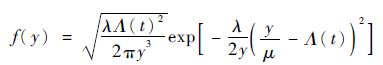(1)

D为产品的失效阈值，则Y(t)首次到达D的时间为产品的寿命ξ=inf{t|Y(t)≥D}ξ的累积分布函数(CDF)可由式(2)推出。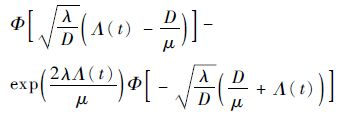(2)

1.2 随机参数Inverse Gaussian过程(3)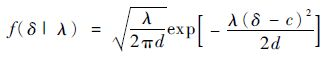(4)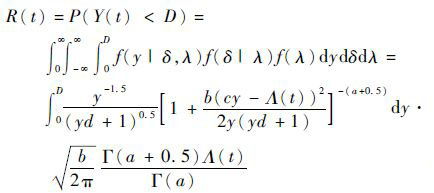(5)
2 加速退化数据折算方法

2.1 推导加速系数表达式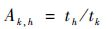(6)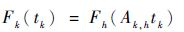(7)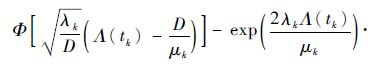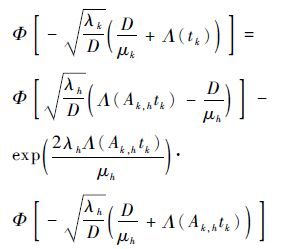(8)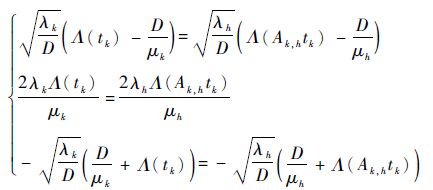(9)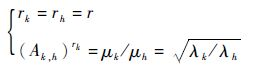(10)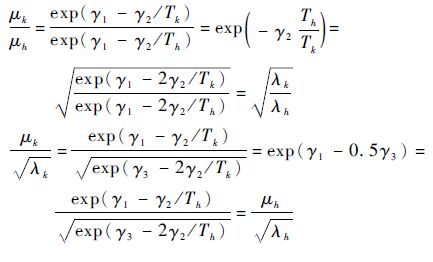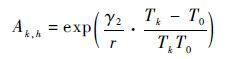(11)
2.2 利用加速系数折算退化数据

T0为产品的工作温度，ti,j,kTk下第i个产品的第j次测量时间,y(ti,j,k)为对应的退化测量值，Λi,j,k=Λ(ti,j,k)-Λ(ti,j-1,k)为时间函数Λ(ti,j,k)的增量,yi,j,k=y(ti,j,k)-y(ti,j-1,k)为退化增量，k=1,2,…,QQ为加速应力总数；i=1,2,…,NkNkTk下的产品数量;j=1,2,…,Mi,kMi,kTk下第i个产品的测量次数。根据Inverse Guassian 分布的统计特性yi,j,k~ IG(exp12/Tii,j,k,exp3-2γ2/Tii,j,k2)，建立似然函数：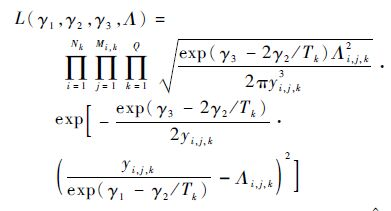(12)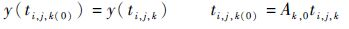(13)

3 参数估计方法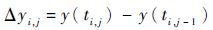为折算后的退化增量，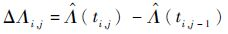为折算后的时间增量，其中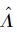可由式(12)估计出。如果能从Δyi,j和ΔΛi,j中估计出未知参数向量 Ω =(a,b,c,d)，就可确定产品的可靠度函数。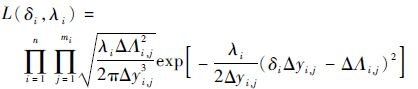(14)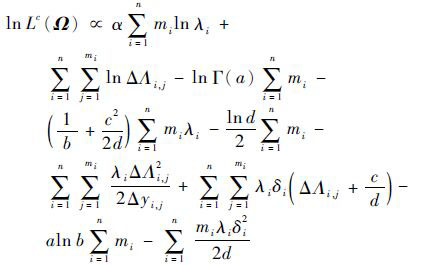(15)

f(δii)为随机参数δi、λi的联合先验概率密度函数，则有f(δii)=f(λi)f(δii)，则联合后验概率密度函数f(δii|Δ y i)，可通过Bayesian公式f(δii|Δ y i)∝L(δii)f(δii)推导出，可得随机参数δi、λi的后验分布为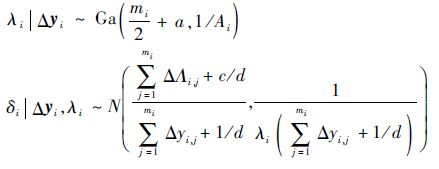(16)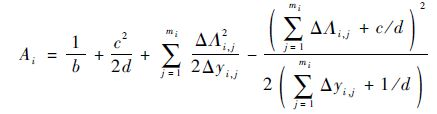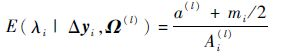(17)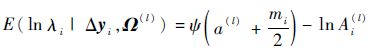(18)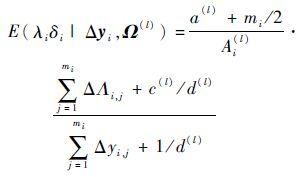(19)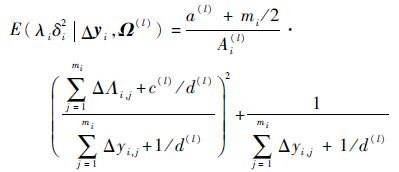(20)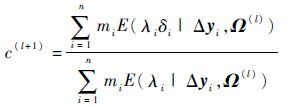(21)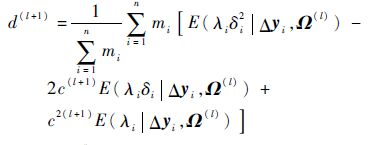(22)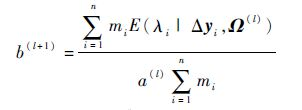(23)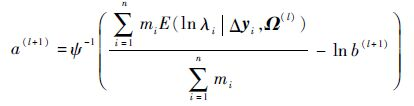(24)

EM算法的执行过程描述如下。

l+1次迭代:

E步:计算E(λi|Δ y i,Ω (l)),E(ln λi|Δ y i,Ω (l)),E(λiδi|Δ y i,Ω (l))E(λiδi2|Δ y i,Ω (l))

M步 : 解得c(l+1)d(l+1)b(l+1)a(l+1)，将 Ω (l)更新为 Ω (l+1)

4 仿真验证 4.1 验证加速系数不变原则的推导结论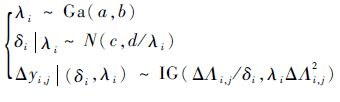(25)

1) 利用仿真模型生成产品在应力Sk下的退化增量数据Δyi,j,k、ΔΛi,j,k

2) 利用Δyi,j,k、ΔΛi,j,k解得Sk下的参数估计值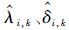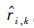3) 分别设加速系数Ak,h0.4,4，根据式(13)计算出折算到Sh下的退化增量数据Δyi,j,h、ΔΛi,j,h

4) 利用Δyi,j,h、ΔΛi,j,h解得Sh下的参数估计值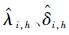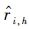5) 计算出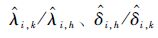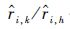平均值，判断是否满足式(9)(其中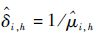)。

 r Ak,h=0.4 Ak,h=4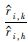的均值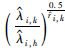的均值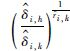的均值的均值的均值的均值 0.5 1.000 0 0.400 1 0.400 1 1.000 0 4.000 2 4.000 1 1.0 1.000 1 0.400 0 0.400 0 1.000 0 4.000 0 4.000 0 2.0 1.000 0 0.400 2 0.400 0 1.000 0 3.999 9 4.000 0

4.2 验证本文所提的建模方法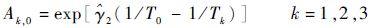(26)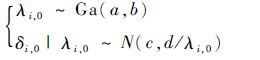(27)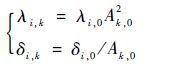(28)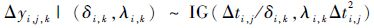(29)

1) 设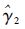=3 000，工作温度T0=400 K，加速温度分别为T1=430 K，T2=460 K，T3=490 K,由式(26)计算出加速系数Ak,0k=1,2,3

2) 设(a,b,c,d)=(2.0,1.0,0.5,0.1)，由式(27)生成T0下的随机参数i,0i,0)i=1,2,…,20

3) 由式(28)生成Tk下的随机参数i,ki,k)k=1,2,3

4) 设∀i,ti,j=1,2,…,10 a，利用i,0i,0)由式(29) 生成T0下的退化增量数据(Δyi,j,0ti,j)

5) 利用i,ki,k)由式(29) 生成Tk下的退化增量数据(Δyi,j,k,Δti,j)k=1,2,3

6) 设失效阈值D=2，利用随机参数Inverse Gaussian模型对(Δyi,j,0ti,j)建模，获得可靠度函数R(0)(t)作为标准值。

7) 利用随机参数Inverse Gaussian模型对(Δyi,j,kti,j)建模，获得可靠度函数R(1)(t)

8) 利用固定参数Inverse Gaussian模型对(Δyi,j,kti,j)建模，获得可靠度函数R(2)(t)

9) 判断R(1)(t)R(2)(t)哪个与R(0)(t)更接近。

R(0)(t)R(1)(t)R(2)(t)的变化曲线如图 1所示，可见R(1)(t)R(0)(t)更接近，说明本文所提的建模方法相比传统的固定参数建模方法提高了可靠性评估的准确性。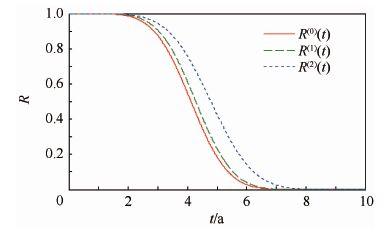图 1 可靠度曲线 Fig. 1 Reliability curves
5 实例应用

Meeker 和 Escobar提供了某型碳膜电阻在恒定应力ADT中的退化数据。3组加速温度应力分别为83、133和173℃，产品的工作温度为50℃，试验过程中所有样品同时测量，测量时刻为452、 1 030、4 341和8 084 h，退化参量为电阻值的百分比增量。 更为详细的试验数据见文献中的Table C.3，因为第27个样品的退化数据不是单调递增，本文剔除了第27个样品的退化数据。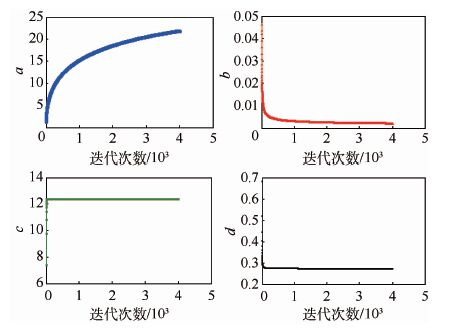图 2 超参数估计值的迭代收敛过程 Fig. 2 Iterative convergence process ofhyper-parameter estimates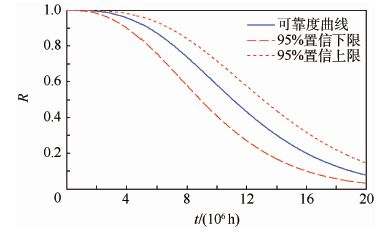图 3 产品在50℃下的可靠度曲线 Fig. 3 Reliability curves of product under 50℃
6 结 论

1) 随机参数Inverse Gaussian过程具备很好的统计特性，适合对严格单调退化过程建模，是除了Wiener过程和Gamma过程之外另一种应用性较好的随机过程模型。

2) 根据加速系数不变原则可推导出退化模型各参数在各加速应力下应满足的关系式，为获得各种退化模型的加速系数表达式提供了一种可行方法。

3) 利用加速系数可实现加速退化数据的等效折算，这种处理思路还可为解决加速退化建模的其他难题提供有益参考。

4) 与传统的基于固定参数的建模方法相比，本文提出的基于随机参数退化模型的建模方法具有更好的拟合效果，能够提高可靠性评估的准确性。

#### 文章信息

WANG Haowei, TENG Kenan, XI Wenjun

Accelerated degradation modeling method based on Inverse Gaussian processes with random parameters

Journal of Beijing University of Aeronautics and Astronsutics, 2016, 42(9): 1843-1850
http://dx.doi.org/10.13700/j.bh.1001-5965.2015.0542